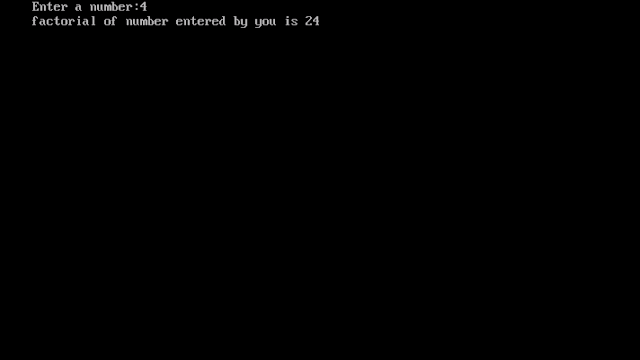# Factorial Program in c using for loop

Factorial program in c: In this program, we are going to find the factorial of any number using for loop.

Enter a number: 8

Factorial of 8 is 40320

## Factorial Program in c using for loop

```#include<stdio.h>
#include<conio.h>
void main()
{
int num,i;
clrscr();
printf("Enter a Number:");
scanf(“%d”,&num);
for(i=num-1;i>=1;i--)
num=num*i;
printf(“Factorial of number”);
printf(“entered by you is %d”,num);
getch();
}```

Output:## Algorithm to make Factorial program in c using for loop

• Initialize an int variable and takes it input from the user using scanf.
• Use for loop, initialize i with the value one less than the number given by the user.
• The testing condition is that our loop variable should be greater than 1.
• The updating expression will be i-- which decreases the value of i by one.
• Now multiply the num variable by our loop variable.
• Now, you can print our variable num which shows the factorial of the number entered by the user.
Factorial:
Factorial is represented by "!".

4! = 4*3*2*1 = 24
n! = n*(n-1)*n-2)*…..*1

## How to Make a Factorial Program in C using for-loop

In this post, I have provided source code and an approach to make a factorial program in c using for loop.

I have a 5-minute task for you today. If you do it, it will take you one step closer to become a programmer.

I know you have already installed turbo c++ or another compiler in your device, but I bet you cannot make a C program to determine the factorial of a number correctly. If you can do so then very well, You can check other programs as well on this site.

So, If you are not able to do so then don't worry about it.  You have to just follow the algorithm and source code. That way you can get a better understanding of what you need to make a factorial program in c using for loop.

Also, this week, I’ve covered a few more lessons when it comes to your Coding training:

Prime number program in c- I break down the source code in many ways like using for-loop, while-loop, do-while loop, and using functions also.

Palindrome program in c- In this lesson, I have given you the source code which helps you to make a program to check whether the number is palindrome or not.

If you have any queries let me know by commenting below.

Hope your programming training is going well😊

Cheers,

CodingWallah• Hackerrank 5* • Full Stack Developer • Masters in SEO

### Related Posts

1.Thank you bhai

2.What is the program of factorial for two digit numbers?

3.This program is also valid for two digit numbers .
No difference Nothing difference

1.But what if I want factorial of only tow digit number?